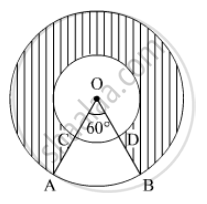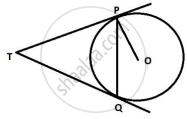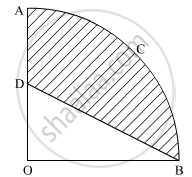# English Medium Class 10 - CBSE Important Questions for Mathematics

Subjects
Topics
Subjects
Popular subjects
Topics
Mathematics
< prev  1 to 20 of 1414  next >

Prove that the lengths of the tangents drawn from an external point to a circle are equal.

Appears in 20 question papers
Chapter: [3.01] Circles
Concept: Number of Tangents from a Point on a Circle

Prove that the tangent at any point of a circle is perpendicular to the radius through the point of contact.

Appears in 14 question papers
Chapter: [3.01] Circles
Concept: Tangent to a Circle

Prove that a parallelogram circumscribing a circle is a rhombus.

Appears in 12 question papers
Chapter: [3.01] Circles
Concept: Number of Tangents from a Point on a Circle

Prove that opposite sides of a quadrilateral circumscribing a circle subtend supplementary angles at the centre of the circle.

Appears in 9 question papers
Chapter: [3.01] Circles
Concept: Number of Tangents from a Point on a Circle

A bucket open at the top is in the form of a frustum of a cone with a capacity of 12308.8 cm3. The radii of the top and bottom circular ends are 20 cm and 12 cm, respectively. Find the height of the bucket and the area of metal sheet used in making the bucket. (use π = 3.14)

Appears in 6 question papers
Chapter: [4.01] Heights and Distances
Concept: Heights and Distances

Prove that the tangents drawn at the ends of a diameter of a circle are parallel.

Appears in 5 question papers
Chapter: [3.01] Circles
Concept: Number of Tangents from a Point on a Circle

In Figure , two concentric circles with centre O, have radii 21cm and 42 cm. If ∠ AOB = 60°, find the area of the shaded region. [use π=22/7]Appears in 5 question papers
Chapter: [7.01] Areas Related to Circles
Concept: Circumference of a Circle

In Figure , two concentric circles with centre O, have radii 21cm and 42 cm. If ∠ AOB = 60°, find the area of the shaded region. [use π=22/7]Appears in 5 question papers
Chapter: [7.01] Areas Related to Circles
Concept: Circumference of a Circle

In an AP of 50 terms, the sum of first 10 terms is 210 and the sum of its last 15 terms is 2565. Find the A.P.

Appears in 4 question papers
Chapter: [2.02] Arithmetic Progressions
Concept: Arithmetic Progression

Solve the following quadratic equation for x: 4x^2 + 4bx – (a^2 – b^2) = 0

Appears in 4 question papers
Concept: Solutions of Quadratic Equations by Completing the Square

A motorboat whose speed is 18 km/hr in still water takes 1 hr more to go 24 km upstream than to return downstream to the same spot. Find the speed of the stream.

Appears in 4 question papers
Chapter: [2.04] Polynomials
Concept: Polynomials

A motorboat whose speed is 18 km/hr in still water takes 1 hr more to go 24 km upstream than to return downstream to the same spot. Find the speed of the stream.

Appears in 4 question papers
Chapter: [2.04] Polynomials
Concept: Polynomials

Two tangents TP and TQ are drawn to a circle with centre O from an external point T. Prove that ∠PTQ = 2∠OPQ.Appears in 4 question papers
Chapter: [3.01] Circles
Concept: Concept of Circle - Centre, Radius, Diameter, Arc, Sector, Chord, Segment, Semicircle, Circumference, Interior and Exterior, Concentric Circles

Two tangents TP and TQ are drawn to a circle with centre O from an external point T. Prove that ∠PTQ = 2∠OPQ.Appears in 4 question papers
Chapter: [3.01] Circles
Concept: Concept of Circle - Centre, Radius, Diameter, Arc, Sector, Chord, Segment, Semicircle, Circumference, Interior and Exterior, Concentric Circles

Construct a triangle ABC with sides BC = 7 cm, ∠B = 45° and ∠A = 105°. Then construct a triangle whose sides are 3/4 times the corresponding sides of ∆ABC.

Appears in 4 question papers
Chapter: [3.02] Triangles
Concept: Application of Pythagoras Theorem in Acute Angle and Obtuse Angle

As observed from the top of a 100 m high lighthouse from the sea-level, the angles of depression of two ships are 30° and 45°. If one ship is exactly behind the other on the same side of the lighthouse, find the distance between the two ships. [Use sqrt3 = 1.732]

Appears in 4 question papers
Chapter: [4.01] Heights and Distances
Concept: Heights and Distances

In the given figure, OACB is a quadrant of a circle with centre O and radius 3.5 cm. If OD = 2 cm, find the area of the shaded region.Appears in 4 question papers
Chapter: [7.01] Areas Related to Circles
Concept: Areas of Sector and Segment of a Circle

Water is flowing at the rate of 2.52 km/h through a cylindrical pipe into a cylindrical tank, the radius of whose base is 40 cm. If the increase in the level of water in the tank, in half an hour is 3.15 m, find the internal diameter of the pipe.

Appears in 4 question papers
Chapter: [7.02] Surface Areas and Volumes
Concept: Concept of Surface Area, Volume, and Capacity

Water is flowing at the rate of 2.52 km/h through a cylindrical pipe into a cylindrical tank, the radius of whose base is 40 cm. If the increase in the level of water in the tank, in half an hour is 3.15 m, find the internal diameter of the pipe.

Appears in 4 question papers
Chapter: [7.02] Surface Areas and Volumes
Concept: Concept of Surface Area, Volume, and Capacity

What is the HCF of the smallest prime number and the smallest composite number?

Appears in 3 question papers
Chapter: [1.01] Real Numbers
Concept: Fundamental Theorem of Arithmetic
< prev  1 to 20 of 1414  next >